# OECD Road accidents data analysis 3 - make a histogram with ggplot() + geom_histogram(). Log conversion makes better looking histogram.Photo by Robert Lukeman on Unsplash

This post is following og above post.

We have five combinations for value,

1. ACCI & NBR

2. DEATH & HAB

3. DEATH & VEH

4. DEATH & NBR

5. INJURE & NBR

So, I will make five sub data frames.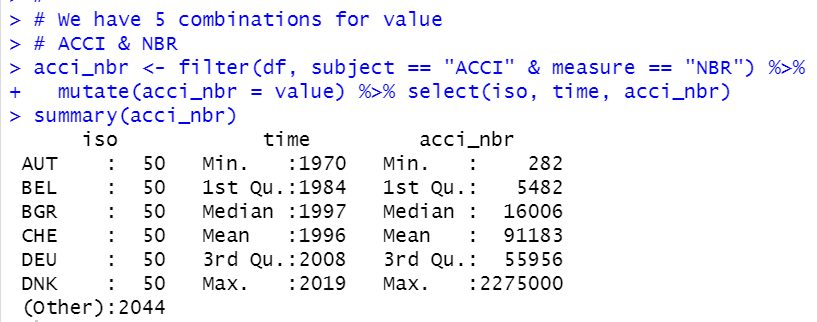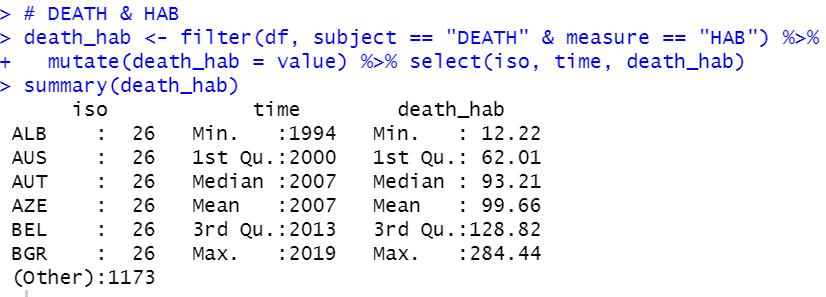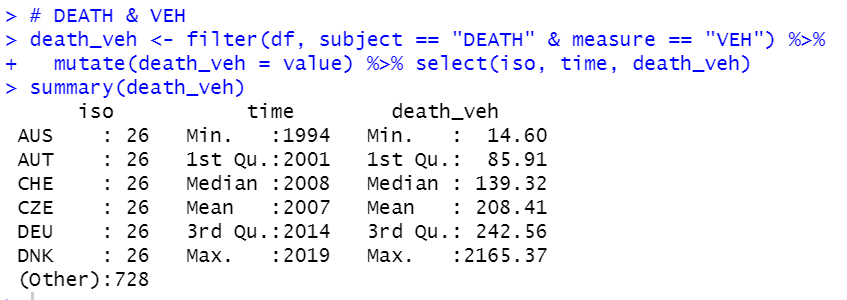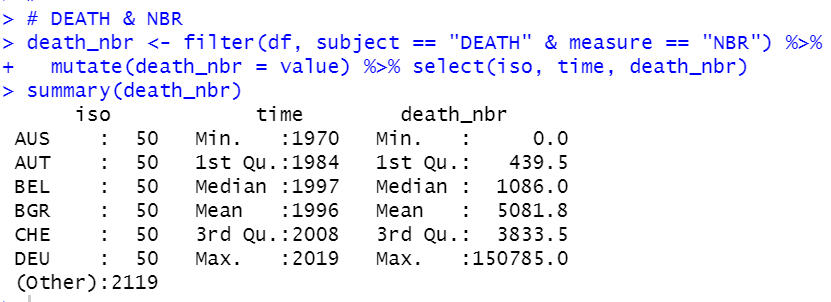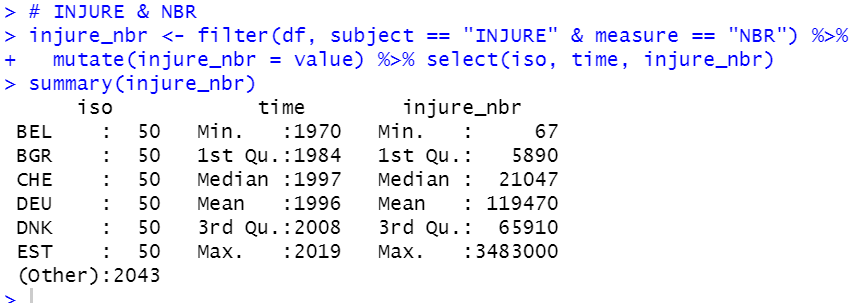Then, let's merge those five data frames.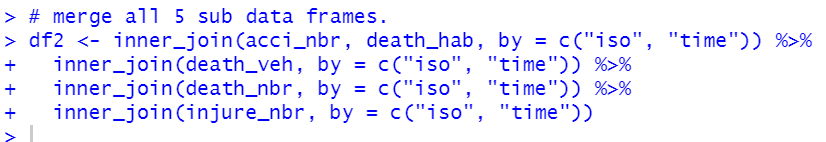I used inner_join() function and common colums are iso and time. So, I add by = c("iso", "time").

Summary is below.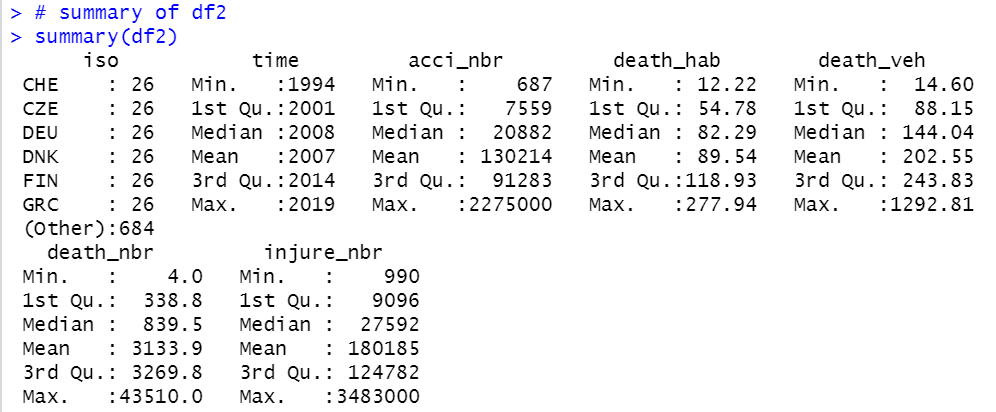We see time starts from 1994 and ends in 2019. It is 26 years.

Let's see each variables histogram.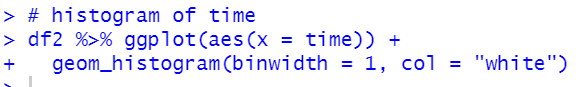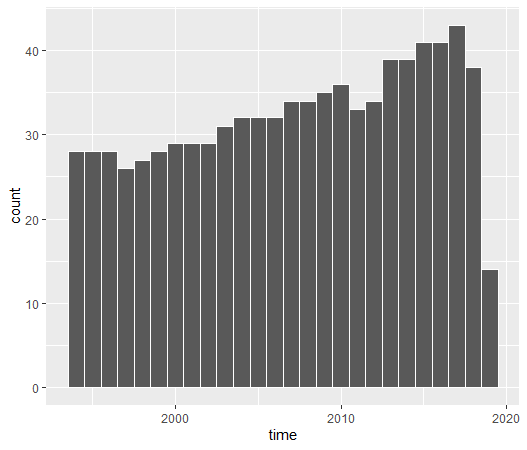We see 2017 has the most observations.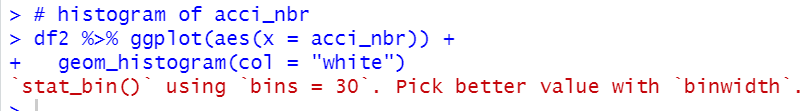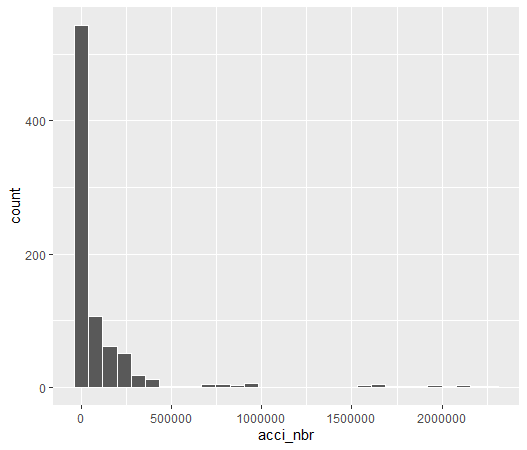For acci_nbr, it is better to convert log value.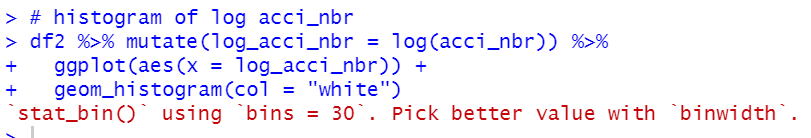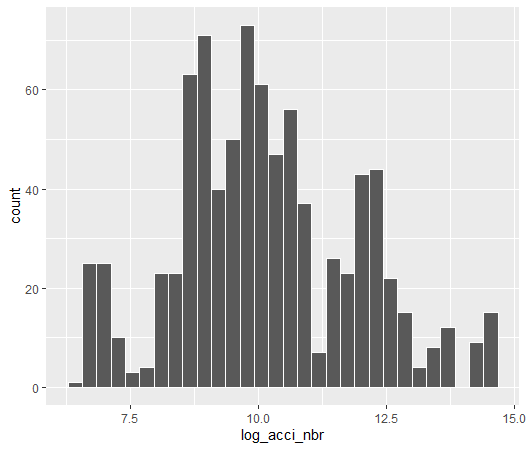Let's see death_hab.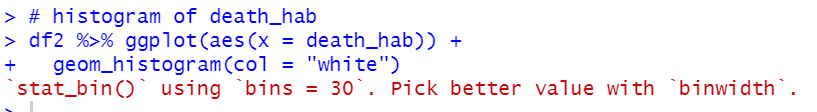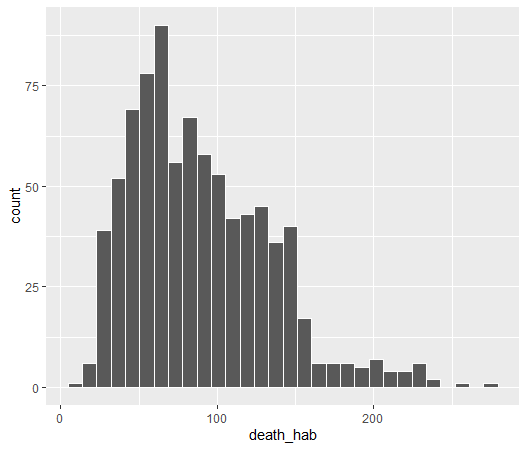It maybe better to convert log value.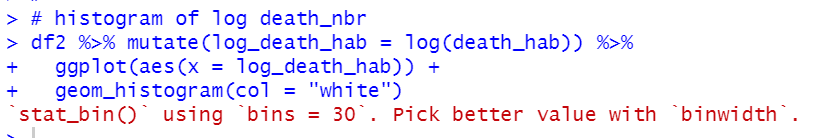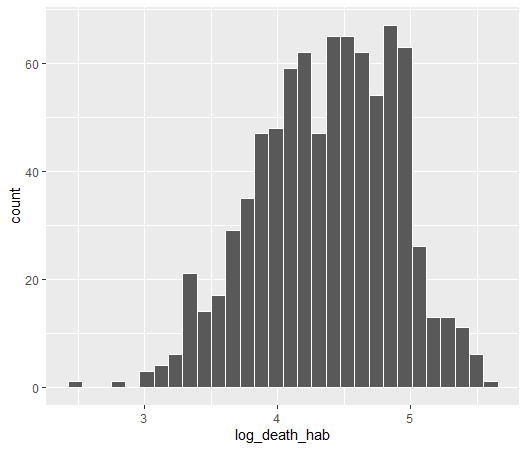Let's see death_veh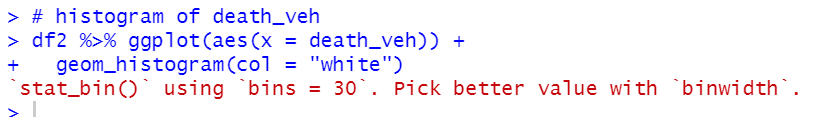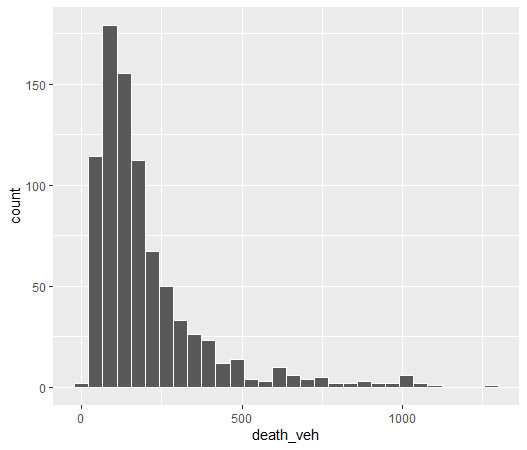It maybe better to convert log value.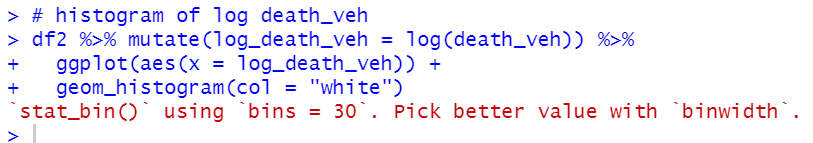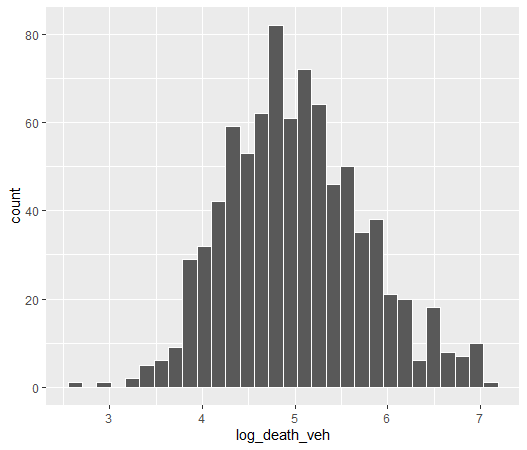Let's see death_nbr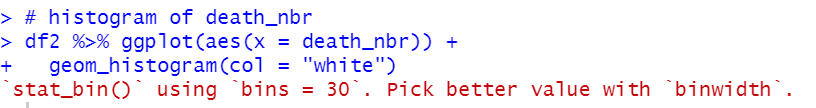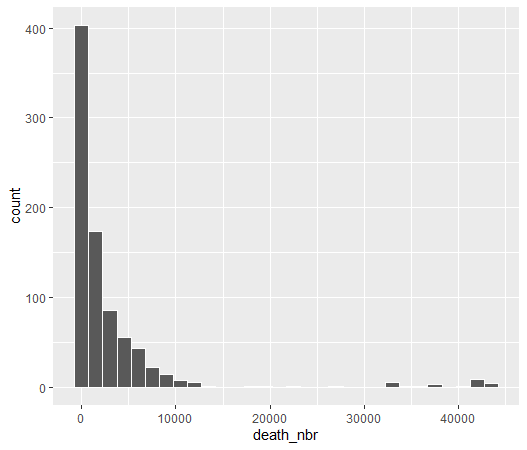It is better to convert log value.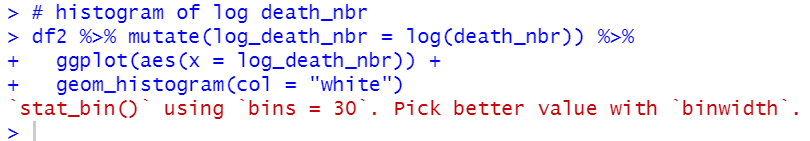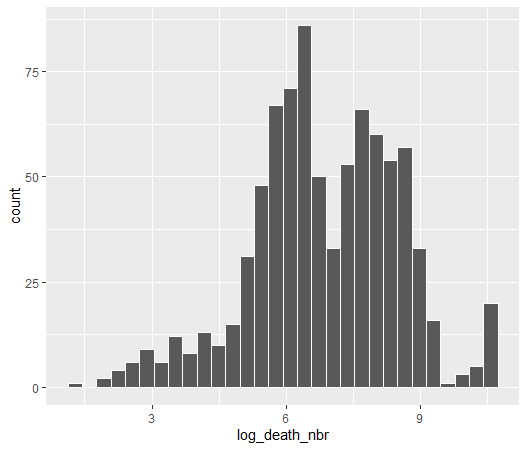Let's see injure_nbr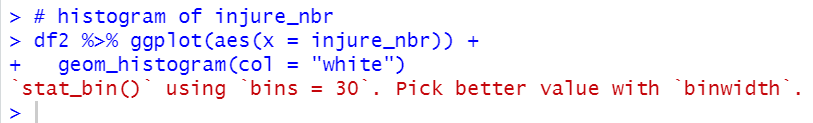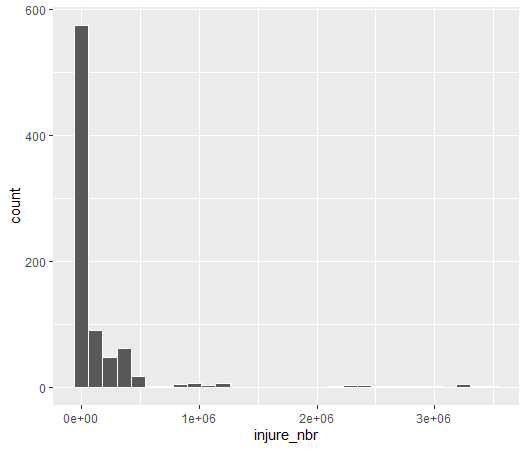It is better to convert log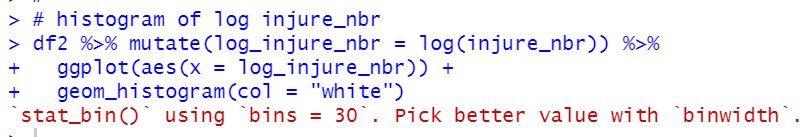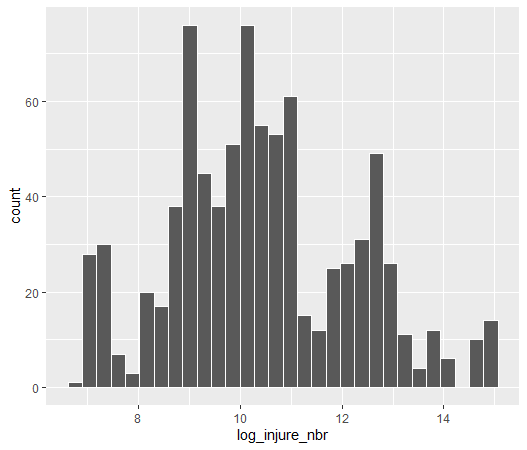We see each variables histogram and found that it is better to convert log value.

That's it. Thank you!

The next post is

To see the 1st post,